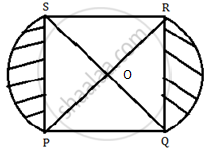# Question Bank Solutions for CBSE Class 10 - CBSE - Mathematics

Subjects
Topics
Subjects
Popular subjects
Topics
Mathematics
< prev 1 to 20 of 8199 next >

Two different dice are tossed together. Find the probability that the product of the two numbers on the top of the dice is 6.

[5.01] Probability
Chapter: [5.01] Probability
Concept: Concept of Probability - Random Experiment, Outcome, Equally Likely Outcomes.

The angle of elevation of an aeroplane from point A on the ground is 60˚. After flight of 15 seconds, the angle of elevation changes to 30˚. If the aeroplane is flying at a constant height of 1500√3 m, find the speed of the plane in km/hr.

[4.01] Heights and Distances
Chapter: [4.01] Heights and Distances
Concept: Heights and Distances

The 13th term of an A.P. is four times its 3rd term. If its 5th term is 16, then find the sum of its first ten terms.

[2.02] Arithmetic Progressions
Chapter: [2.02] Arithmetic Progressions
Concept: Arithmetic Progression

A bag contains, white, black and red balls only. A ball is drawn at random from the bag.If the probability of getting a white ball is 3/10 and that of a black ball is 2/5 then find the
probability of getting a red ball. If the bag contains 20 black balls, then find the total number of balls in the bag.

[5.01] Probability
Chapter: [5.01] Probability
Concept: Concept of Probability - Random Experiment, Outcome, Equally Likely Outcomes.

At a point A, 20 metres above the level of water in a lake, the angle of elevation of a cloud is 30˚. The angle of depression of the reflection of the cloud in the lake, at A is 60˚.
Find the distance of the cloud from A.

[4.01] Heights and Distances
Chapter: [4.01] Heights and Distances
Concept: Heights and Distances

A card is drawn at random from a well-shuffled deck of playing cards. Find the probability that the card drawn is a card of spade or an ace.

[5.01] Probability
Chapter: [5.01] Probability
Concept: Concept of Probability - Random Experiment, Outcome, Equally Likely Outcomes.

In the figure given below, PQRS is square lawn with side PQ = 42 metres. Two circular flower beds are there on the sides PS and QR with centre at O, the intersections of its
diagonals. Find the total area of the two flower beds (shaded parts).[7.01] Areas Related to Circles
Chapter: [7.01] Areas Related to Circles
Concept: Circumference of a Circle

Prove that the tangent drawn at the mid-point of an arc of a circle is parallel to the chord joining the end points of the arc.

[3.01] Circles
Chapter: [3.01] Circles
Concept: Concept of Circle - Centre, Radius, Diameter, Arc, Sector, Chord, Segment, Semicircle, Circumference, Interior and Exterior.

A truck covers a distance of 150 km at a certain average speed and then covers another 200 km at an average speed which is 20 km per hour more than the first speed. If the truck covers the total distance in 5 hours, find the first speed of the truck.

[4.01] Heights and Distances
Chapter: [4.01] Heights and Distances
Concept: Heights and Distances

An arithmetic progression 5, 12, 19, …. has 50 terms. Find its last term. Hence find the sum of its last 15 terms.

[2.02] Arithmetic Progressions
Chapter: [2.02] Arithmetic Progressions
Concept: Arithmetic Progression

Construct a triangle ABC in which AB = 5 cm, BC = 6 cm and ∠ABC = 60˚. Now construct another triangle whose sides are 5/7 times the corresponding sides of ΔABC.

[3.03] Constructions
Chapter: [3.03] Constructions
Concept: Division of a Line Segment

The probability of selecting a red ball at random from a jar that contains only red, blue and  orange balls is 1/4. The probability of selecting a blue ball at random from the same jar 1/3. If the jar contains 10 orange balls, find the total number of balls in the jar.

[5.01] Probability
Chapter: [5.01] Probability
Concept: Concept of Probability - Random Experiment, Outcome, Equally Likely Outcomes.

If the coordinates of points A and B are (-2, -2) and (2, -4) respectively, find the coordinates of P such that AP =(3/7)AB, where P lies on the line segment AB.

[3.03] Constructions
Chapter: [3.03] Constructions
Concept: Division of a Line Segment

All the vertices of a rhombus lie on a circle. Find the area of the rhombus, if the area of the circle is 1256 cm2. [Use p = 3.14]

[7.01] Areas Related to Circles
Chapter: [7.01] Areas Related to Circles
Concept: Circumference of a Circle

The 16th term of an A.P. is five times its third term. If its 10th term is 41, then find the sum of its first fifteen terms.

[2.02] Arithmetic Progressions
Chapter: [2.02] Arithmetic Progressions
Concept: Arithmetic Progression

Find the 60th term of the A.P. 8, 10, 12, ……., if it has a total of 60 terms and hence find the sum of its last 10 terms.

[2.02] Arithmetic Progressions
Chapter: [2.02] Arithmetic Progressions
Concept: Arithmetic Progression

A bus travels at a certain average speed for a distance of 75 km and then travels a distance of 90 km at an average speed of 10 km/h more than the first speed. If it takes 3 hours to complete the total journey, find its first speed?

[4.01] Heights and Distances
Chapter: [4.01] Heights and Distances
Concept: Heights and Distances

The 14th term of an A.P. is twice its 8th term. If its 6th term is -8, then find the sum of its first 20 terms.

[2.02] Arithmetic Progressions
Chapter: [2.02] Arithmetic Progressions
Concept: Arithmetic Progression

Construct a Δ ABC in which AB = 6 cm, ∠A = 30° and ∠B = 60°, Construct another ΔAB’C’ similar to ΔABC with base AB’ = 8 cm.

[3.03] Constructions
Chapter: [3.03] Constructions
Concept: Division of a Line Segment

The probability that a number selected at random from the numbers 1, 2, 3, ..., 15 is a multiple of 4, is

(A)4/15

(B)2/15

(C)1/5

(D)1/3

[5.01] Probability
Chapter: [5.01] Probability
Concept: Concept of Probability - Random Experiment, Outcome, Equally Likely Outcomes.
< prev 1 to 20 of 8199 next >